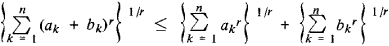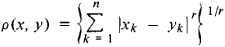# Minkowski Inequality

(redirected from Minkowski inequalities)
The following article is from The Great Soviet Encyclopedia (1979). It might be outdated or ideologically biased.

## Minkowski Inequality

an inequality of the formwhere ak and ak (k = 1,2, . . . , n) are non-negative numbers and r > 1. The Minkowski inequality has analogs for infinite series and integrals. The inequality was established by H. Minkowski in 1896 and expresses the fact that in n-dimensional space, where the distance between the points x = (x1, x2, . . . , xn) and y = (y1, y2, . . . , yn) is given bythe sum of the lengths of two sides of a triangle is greater than the length of the third side.

The Great Soviet Encyclopedia, 3rd Edition (1970-1979). © 2010 The Gale Group, Inc. All rights reserved.
Site: Follow: Share:
Open / Close